# WA Inverse Integer Wavelet Transform VI

LabVIEW 2014 Advanced Signal Processing Toolkit Help

Edition Date: June 2014

Part Number: 372656C-01

»View Product InfoDownload Help (Windows Only)

Owning Palette: Discrete Wavelet VIs

Computes the multi-level inverse integer wavelet transform (IWT) and returns the reconstructed signal from the approximation coefficients and the detail coefficients. Wire data to the IWT coef input to determine the polymorphic instance to use or manually select the instance.

Examples

Use the pull-down menu to select an instance of this VI.

 Select an instance WA Inverse 1D Integer Wavelet TransformWA Inverse 2D Integer Wavelet Transform

## WA Inverse 1D Integer Wavelet Transform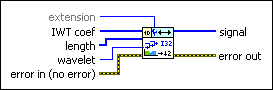extension specifies the method to use to pad data at the borders of the approximation coefficients and the detail coefficients before each iteration of the lifting scheme. The coefficient extension is essential for reconstructing the entire signal. When you select the extension method, make the transition between the input signal and the padded data as smooth as possible because a smooth transition generates fewer large detail coefficients and enhances the efficiency of the signal representation.

 0 Zero padding—Uses zeros to pad the input data. Watch for abrupt transitions between the padded zeros and the input data, which causes large artifacts near the transition. 1 Symmetric—Uses replications of the input data to pad the data, except that this VI left-flips the block at the input and right-flips the block at the end. 2 Periodic (default)—Adds a replication of the input data block before and another replication after the input data block to pad the data.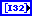IWT coef specifies the approximation coefficients and the detail coefficients for the multi-level inverse integer wavelet transform (IWT). You must organize the coefficients into a 1D integer array starting with the approximation coefficients at the largest level followed by the detail coefficients at all levels in descending order.length specifies the length of the approximation coefficients, the detail coefficients, and the signal. You must organize length into a 1D array starting with the length of the approximation coefficients at the largest level, the length of the detail coefficients at all levels in descending order, and the length of the signal.wavelet specifies the wavelet type of the integer wavelet analysis. The default is Haar. wavelet must be the same type as the wavelet type you selected in the WA Integer Wavelet Transform VI to compute IWT coef.error in describes error conditions that occur before this node runs. This input provides standard error in functionality.signal returns the reconstructed integer signal.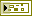error out contains error information. This output provides standard error out functionality.

## WA Inverse 2D Integer Wavelet Transform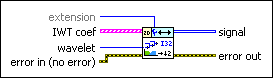extension specifies the method to use to pad data at the borders of the approximation coefficients and the detail coefficients before each iteration of the lifting scheme. The coefficient extension is essential for reconstructing the entire signal. When you select the extension method, make the transition between the input signal and the padded data as smooth as possible because a smooth transition generates fewer large detail coefficients and enhances the efficiency of the signal representation.

 0 Zero padding—Uses zeros to pad the input data. Watch for abrupt transitions between the padded zeros and the input data, which causes large artifacts near the transition. 1 Symmetric—Uses replications of the input data to pad the data, except that this VI left-flips the block at the input and right-flips the block at the end. 2 Periodic (default)—Adds a replication of the input data block before and another replication after the input data block to pad the data.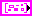IWT coef specifies the approximation coefficients and the detail coefficients for the multi-level inverse integer wavelet transform (IWT). Each element of the array contains the 2D IWT results of one level. The ith element stores the approximation coefficients and the detail coefficients at level i+1.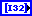low_low specifies the coefficients that are a low-resolution approximation of the original 2D signal.low_high specifies the low_high coefficients. The high-frequency signal along the column direction influences the low_high coefficients.high_low specifies the high_low coefficients. The high-frequency signal along the row direction influences the high_low coefficients.high_high specifies the high_high coefficients. The high-frequency signal along the diagonal direction influences the high_high coefficients.wavelet specifies the wavelet type of the integer wavelet analysis. The default is Haar. wavelet must be the same type as the wavelet type you selected in the WA Integer Wavelet Transform VI to compute IWT coef.error in describes error conditions that occur before this node runs. This input provides standard error in functionality.signal returns the reconstructed integer signal.error out contains error information. This output provides standard error out functionality.

## Examples

Refer to the following VIs for examples of using the WA Inverse Integer Wavelet Transform VI:

• Lossless Medical Image Compression VI: labview\examples\Wavelet Analysis\WAApplications
• Integer Wavelet Transform on Image VI: labview\examples\Wavelet Analysis\WAGettingStarted
• Integer Wavelet Transform on 1D Signal VI: labview\examples\Wavelet Analysis\WAGettingStarted